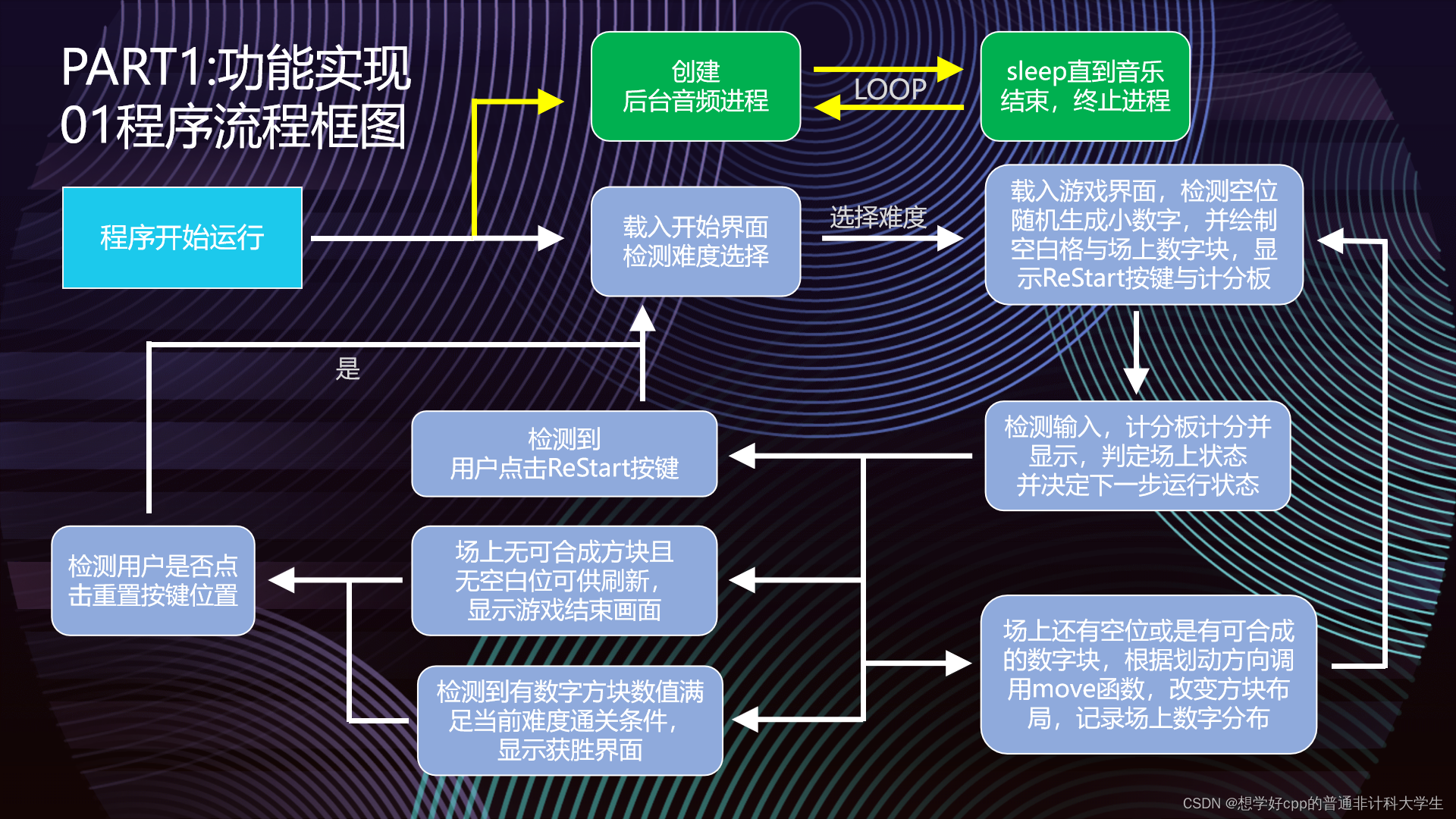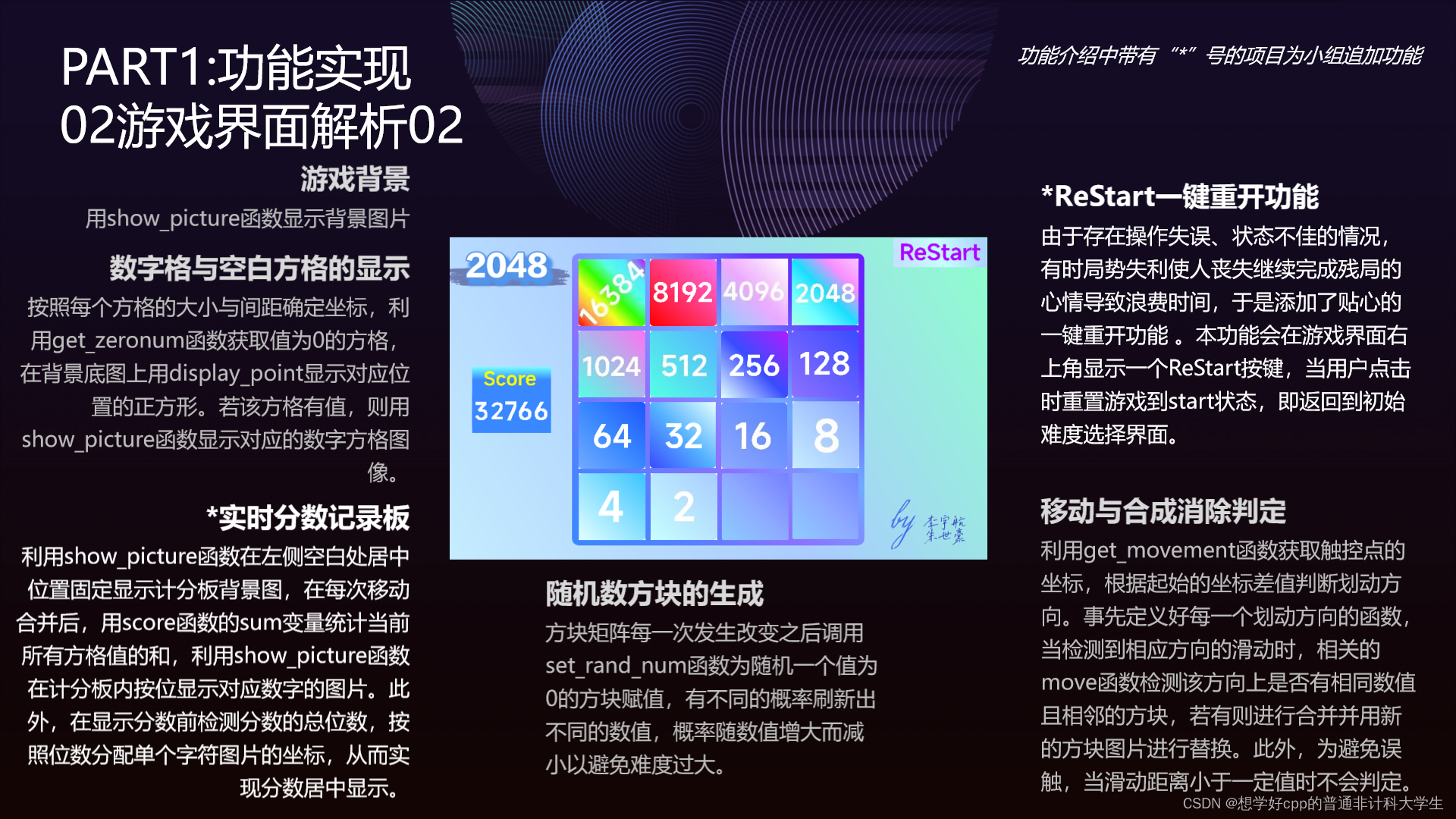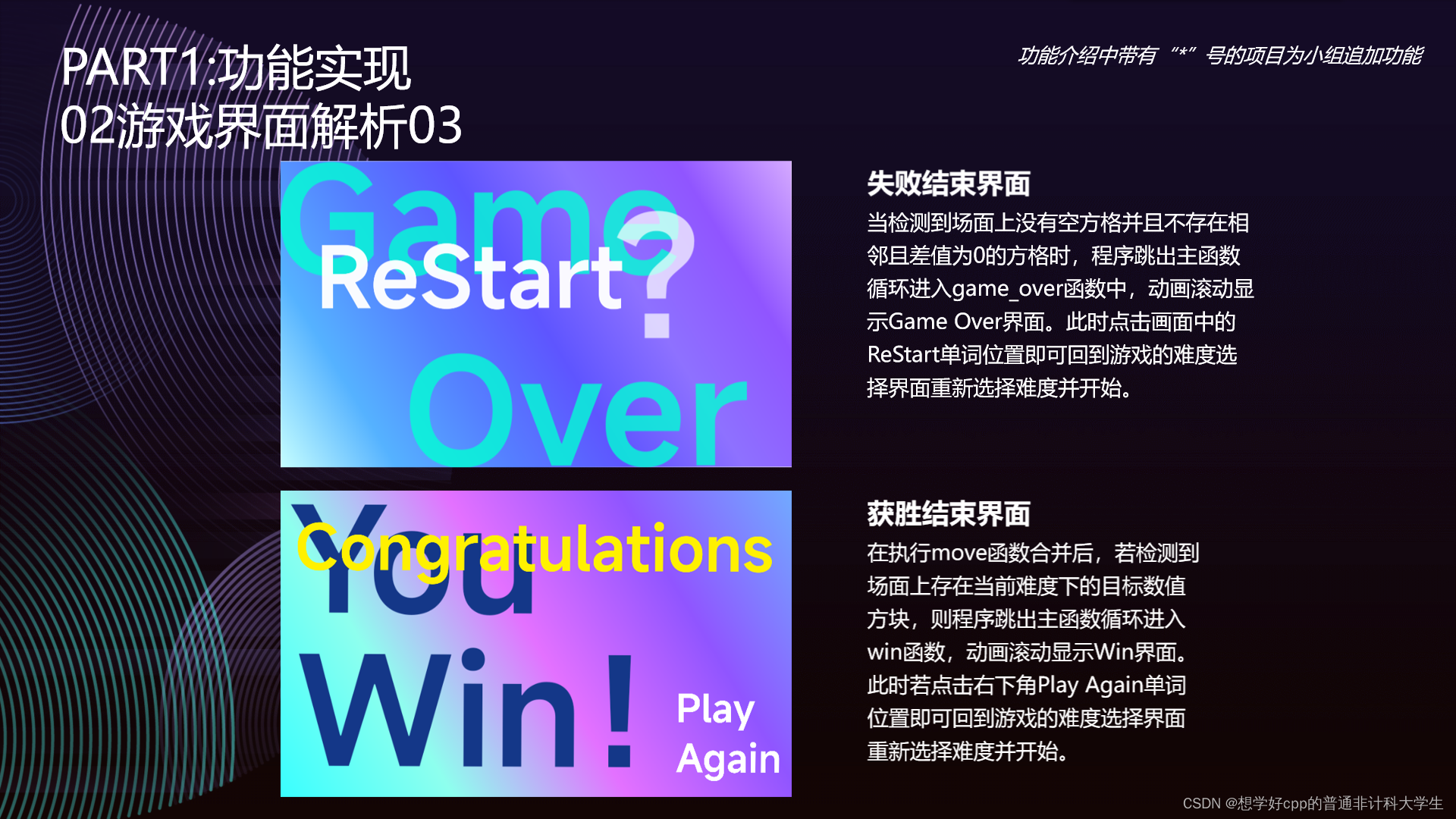# 基于6818粤嵌开发板的2048游戏项目

### 一、lcd.c代码

#### lcd屏幕初始化

// lcd屏幕的初始化
// 打开屏幕
int lcd_init(){
lcd_fd = open( "/dev/fb0" , O_RDWR );
// 判断打开是否成功
if( lcd_fd == -1 ){
// 返回打开失败的原因
perror("open failed!");
return -1;
}
// 内存映射
// MaxSize = 800 * 480
plcd = mmap( NULL , MaxSize*4 , PROT_READ | PROT_WRITE , MAP_SHARED , lcd_fd , 0 );
return 0;
}

#### 在lcd屏幕上任意一点显示颜色

屏幕左上角为( 0 , 0 )，右下角为( lcd_width-1 , lcd_height-1 )。

// 因为是从0开始的，所以最后一个是n-1

其中屏幕上我们用到的是指针，所以随机一点位置为( x , y )的话，那么其地址就为

首地址+x+y*lcd_width.

首地址为我们映射所得得返回值plcd，所以随机一点地址为plcd+x+y*lcd_width.

// 在任意的点上 显示任意的一个颜色
void display_point(int x, int y, int color)
{
if( x >= 0 && x < 800 && y >= 0 && y < 480 )
*(plcd + x + y*800) = color;
}

#### 关闭lcd屏幕

第一个为文件，第二个为字节大小。

// 关闭屏幕
int lcd_close()
{
// 关闭文件
close(lcd_fd);
// 解除映射
// Maxsize = 800 * 480
int res = munmap( plcd , MaxSize*4 );
if( res == -1 ){
perror("Removal failed!");
return -1;
}
return 0;
}


#### lcd.h

#ifndef __LCD_H__
#define __LCD_H__

// lcd屏幕的初始化
int lcd_init();

// 在任意的点上 显示任意的一个颜色
void display_point(int x, int y, int color);

// 关闭屏幕
int lcd_close();

#endif

### 二、bmp.c代码

#### bmp格式

bmp文件时有固定格式的，我们需要了解其格式，才能准确的读取到图片的数据

/*
* 00-01 文件标识，为字母ASCII码"BM"					2byte
* 02-05 文件大小									    4byte
* 06-09 位图文件保留字，必须为0 					    4byte
* 0A-0D 文件开始到位图数据开始之间的偏移量			    4byte
* 0E-11 图像描述信息块的大小，常为28H				    4byte
* 12-15 图片高度									    4byte
* 16-19 图片宽度 									4byte
* 1A-1B 图像plane总数，恒为 1						2byte
* 1C-1D 记录颜色的位数								2byte
* 1E-21 数据压缩方式								    2byte
* 22-25 图像区数据大小，必须为4的倍数				    4byte
* 26-29 水平像素点个数（在设备无关位图中，00H）		4byte
* 2A-2D 垂直像素点个数 （在设备无关位图中，00H）	    4byte
* 2E-31 图像所用颜色数（不用，固定为0）				4byte
* 32-35 重要颜色数（不用，固定为0）					4byte
*
*/

#### bmp图片读取

// 显示图片
void show_picture(char * pathname ,int x ,int y)
{
int fd = open(pathname,O_RDONLY);
if(fd == -1)
{
perror("open error\n");
return ;
}

int width,height;
short depth;
unsigned char buf ;
//读取宽度
lseek(fd,0x12,SEEK_SET);
width = buf<<24 | buf<< 16 | buf << 8 | buf;
//读取高度
height  = buf<<24 | buf<< 16 | buf << 8 | buf;
//读取色深
lseek(fd,0x1c,SEEK_SET);
depth = buf << 8  | buf;
//像素数组
int line_valid_bytes = abs(width) * depth / 8 ; //一行本有的有效字节
int laizi = 0; //填充字节， 文件大小为 54 + wedth*height + height*n 为4的倍数
if( (line_valid_bytes % 4) !=0   ) laizi =  4 - line_valid_bytes%4;
int line_bytes = line_valid_bytes + laizi; //一行所有的字节数
int total_bytes = line_bytes * abs(height); //整个像素数组的大小
unsigned char * p1  = malloc(total_bytes); // 获取动态数组

// 像素为54字节之后，所以调到54读完
lseek(fd,54,SEEK_SET);

// 画点，画图
unsigned char a ,r ,g, b ;
int i = 0;//用来做指针运动的
int x0=0,y0=0; //用来循环计数
int color;
for( y0 = 0 ; y0 < abs(height) ; y0 ++ ) { // 列
for( x0 = 0 ; x0 < abs(width) ; x0 ++ ) { // 行
//一字节一字节读入RGBA
// 读取后，图片顺序会反过来，需要调整
b = p1[i++];
g = p1[i++];
r = p1[i++];
if(depth == 32)
{
a=p1[i++];
}
if(depth == 24)
{
a = 0;
}
color = a << 24 | r << 16 | g << 8 | b ;
// 描绘该点
display_point(width>0?x+x0:abs(width)+x-1-x0, height>0? y+height-1-y0 : y+y0,color);

}
// 一行弄完需要进行填充过滤
i = i +laizi;
}
// 释放指针
free(p1);
close(fd);
}

#### bmp.h

#ifndef __BMP_H__
#define __BMP_H__

// 显示图片
void show_picture( char* pathname , int x , int y );

#endif

### 三、work.c部分源码

#### 触摸屏

// 移动
int get_movement(){
int fd = open("/dev/input/event0", O_RDONLY);
int res;
if (fd == -1) {
printf("open /dev/event0 failed\n");
return -1;
}
int x1 = -1, y1 = -1; // 接触时候的坐标点
int x2, y2; // 离开后的坐标点
struct input_event ev;
while( 1 ){
res = read( fd , &ev, sizeof(ev) );
if( res != sizeof(ev) ) continue;
if( ev.type == EV_ABS && ev.code == ABS_X ) {
if( x1 == -1 ) x1 = ev.value;
x2 = ev.value;
}
if (ev.type == EV_ABS && ev.code == ABS_Y) {
if (y1 == -1) y1 = ev.value;
y2 = ev.value;
}
if( (ev.type == EV_ABS && ev.code == ABS_PRESSURE && ev.value == 0 ) ||
( ev.type == EV_KEY && ev.code == BTN_TOUCH && ev.value == 0 ) ) {

printf("x2 = %d\ty2 = %d\n",x2,y2);
printf("flag = %d\n",flag);
printf("\n\n\n\n");
if( flag ) return 0;
printf("x1 = %d\ty1 = %d\nx2 = %d\ty2 = %d\n",x1,y1,x2,y2);
int opposite_x = abs(x2-x1); // 左右
int opposite_y = abs(y2-y1); // 上下
printf("opposite_x = %d\topposite_y = %d\n",opposite_x,opposite_y);

if( x2 >= 830 && y2 < 80 && opposite_x <= 40 && opposite_y <= 32 ) {
flag = 1;
return 0;
}
if( opposite_x <= 40 && opposite_y <= 32 ) {
x1 = -1;
y1 = -1;
continue;
}
if( opposite_x > 2 * opposite_y ){ // 左右移动
if( x2 > x1 ){ // 方块向右边移动
close(fd);
return MOVE_RIGHT;
}
else{ // 方块向左边移动
close(fd);
return MOVE_LEFT;
}
}
else if( opposite_x < 2* opposite_y ){ // 上下移动
if( y2 > y1 ){ // 方块向下移动
close(fd);
return MOVE_DOWN;
}
else{ // 方块向上移动
close(fd);
return MOVE_UP;
}
}
else x1 = -1, y1 = -1;
}
}
close(fd);
}

#### 2048方块移动算法

2048是一个4*4的矩阵，滑动可以将方块移动并且合并，然后结束一次滑动后可以生成一个新的数字，可能是2或者4。

// 向上移动
void move_up(){
int i, j;
int x, y;
for (i = 0; i < ITEM_NUM; i++) {
for (x = 0; x < ITEM_NUM; ) {
if (matrix_2048[x][i] != 0) {
for (y = x + 1; y < ITEM_NUM; y++) {
if (matrix_2048[y][i] != 0) {
if (matrix_2048[x][i] == matrix_2048[y][i]) {
matrix_2048[x][i] += matrix_2048[y][i];
matrix_2048[y][i] = 0;
x = y + 1;
break;
}
else x = y;
}
}
if (y >= ITEM_NUM) break;
}
else x++;
}
x = 0;
for (y = 0; y < ITEM_NUM; y++) {
if (matrix_2048[y][i] != 0) {
if (x != y) {
matrix_2048[x][i] = matrix_2048[y][i];
matrix_2048[y][i] = 0;
}
x++;
}
}
}
}

#### 在随机位置上生成2或者4

int get_zeronum(){
int i, j, n = 0;
for( i = 0 ; i < ITEM_NUM ; i ++ )
for( j = 0 ; j < ITEM_NUM ; j ++ )
if( matrix_2048[i][j] == 0 )
n ++;
return n;
}

void set_rand_num(){
int zero_Num = get_zeronum();
int n = 0;
int pos = rand() % zero_Num; // pos为随机出现2的位置   pos -> [0,zero_Num) 整数
int i, j;
for( i = 0 ; i < ITEM_NUM ; i ++ )
for( j = 0 ; j < ITEM_NUM ; j ++ )
sum += matrix_2048[i][j];

for( i = 0 ; i < ITEM_NUM ; i ++ )
for( j = 0 ; j < ITEM_NUM ; j ++ )
if( matrix_2048[i][j] == 0 ){
if( n == pos ) {
if( sum >=    2200 ) {
int res = rand()%100000;
if( res == 99999 ) {
matrix_2048[i][j] = 16384;
return;
}
}
int res = rand()%3;
if( res < 2 ) matrix_2048[i][j] = 2;
else matrix_2048[i][j] = 4;
return;
}
else n++;
}
}

#### 绘出4*4矩阵

void LCD_draw_matrix(){
int i, j;
int x0, y0;
for( i = 0 ; i < ITEM_NUM ; i ++ ){
for( j = 0 ; j < ITEM_NUM ; j ++ ){
x0 = MATRIX_X0 + ( ITEM_WIDTH + BLACK_LINE ) * j + 5;
y0 = MATRIX_Y0 + ( ITEM_HEIGHT + BLACK_LINE ) * i + 5;
if( matrix_2048[i][j] == 0 ) {
int k, z;
for( k = 0 ; k < ITEM_WIDTH ; k ++ )
for( z = 0 ; z < ITEM_HEIGHT ; z ++ )
display_point( x0 + k , y0 + z , 0x4682b4 );
}
else{
// 加载那个数字的图片
char pathname;
sprintf( pathname , "%d.bmp" , matrix_2048[i][j] );
show_picture( pathname , x0 , y0 );
}
}
}
}

### 四、流程框图和界面功能描述2048游戏项目功能

04-25
06-30102
06-25
06-14429
06-183717
04-216599
07-091万+
11-171万+
08-131911
03-121万+
05-283785

### “相关推荐”对你有帮助么？

•非常没帮助
•没帮助
•一般
•有帮助
•非常有帮助被折叠的  条评论 为什么被折叠?到【灌水乐园】发言¥1 ¥2 ¥4 ¥6 ¥10 ¥20获取中扫码支付点击重新获取扫码支付1.余额是钱包充值的虚拟货币，按照1:1的比例进行支付金额的抵扣。
2.余额无法直接购买下载，可以购买VIP、付费专栏及课程。余额充值